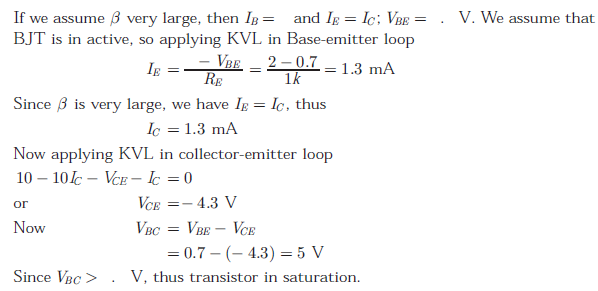# EC GATE 2007 - Online Test

Q1. If R(τ) is the auto correlation function of a real, wide-sense stationary random process, then which of the following is NOT true
Explaination / Solution:
No Explaination.

Q2. A plane wave of wavelength λ is traveling in a direction making an angle 300 with positive x − axis and 900 with positive y − axis. The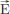field of the plane wave can be represented as (E is constant)
Explaination / Solution: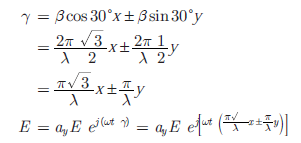Q3. A p n junction has a built-in potential of 0.8 V. The depletion layer width a reverse bias of 1.2 V is 2 μm. For a reverse bias of 7.2 V, the depletion layer width will be
Explaination / Solution: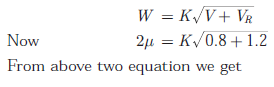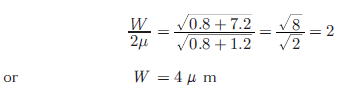Q4. If S(f) is the power spectral density of a real, wide-sense stationary random process, then which of the following is ALWAYS true?
Explaination / Solution:
No Explaination.

Q5. Group I lists four types of p − n junction diodes. Match each device in Group I with one of the option in Group II to indicate the bias condition of the device in its normal mode of operation. Group - I Group-II (P) Zener Diode (1) Forward bias (Q) Solar cell (2) Reverse bias (R) LASER diode (S) Avalanche Photodiode
Explaination / Solution:

Zener diode and Avalanche diode works in the reverse bias and laser diode works in forward bias. In solar cell diode works in forward bias but photo current is in reverse direction. Thus Zener diode : Reverse Bias Solar Cell : Forward Bias Laser Diode : Forward Bias Avalanche Photo diode : Reverse Bias

Q6. In delta modulation, the slope overload distortion can be reduced by
Explaination / Solution:
No Explaination.

Q7. The DC current gain (β) of a BJT is 50. Assuming that the emitter injection efficiency is 0.995, the base transport factor is
Explaination / Solution:Q8.
Group I lists four different semiconductor devices. match each device in Group I with its charactecteristic property in Group II
Group-I                                      Group-II
(P) BJT                                       (1) Population iniversion
(Q) MOS capacitor                     (2) Pinch-off voltage
(R) LASER diode                        (3) Early effect
(S) JFET                                     (4) Flat-band voltage
Explaination / Solution:

In BJT as the B-C reverse bias voltage increases, the B-C space charge region width increases which xB (i.e. neutral base width) > A change in neutral base width will change the collector current. A reduction in base width will causes the gradient in minority carrier concentration to increase, which in turn causes an increased in the diffusion current. This effect si known as base modulation as early effect.
In JFET the gate to source voltage that must be applied to achieve pinch off voltage is described as pinch off voltage and is also called as turn voltage or threshold voltage.
In LASER population inversion occurs on the condition when concentration of electrons in one energy state is greater than that in lower energy state, i.e. a non equilibrium condition.
In MOS capacitor, flat band voltage is the gate voltage that must be applied to create flat ban condition in which there is no space charge region in semiconductor under oxide.
Therefore
BJT : Early effect
MOS capacitor : Flat-band voltage
LASER diode : Population inversion
JFET : Pinch-off voltage

Q9. The raised cosine pulse p(t) is used for zero ISI in digital communications. The expression for p(t) with unity roll-off factor is given byThe value of p(t) at t = 1/W is
Explaination / Solution: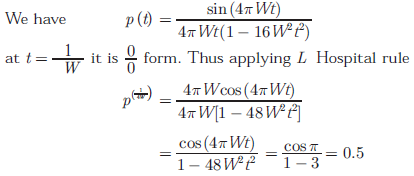Q10. For the BJT circuit shown, assume that the β of the transistor is very large and VBE = . V. The mode of operation of the BJT isExplaination / Solution: